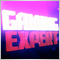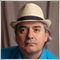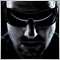# I want the double value in decimal form787

I want the double value in decimal form like 0.000000000114545(this is a random digit)

instead of 6.0050052868833982e-05 because the actual number in decimal is extremely small but i need decimal

Note = These 2 numbers are not equal instead just random examples. do not execute commands on these numbers.

can someone please help me ?6469

Kailash Mina: I want the double value in decimal form like 0.000000000114545(this is a random digit) instead of 6.0050052868833982e-05 because the actual number in decimal is extremely small but i need decimal. Note = These 2 numbers are not equal instead just random examples. do not execute commands on these numbers. can someone please help me ?

You only see that difference when you output the values, or convert to strings, so use "StringFormat()" or "PrintFormat()" to control how you want them formatted.

You can also set the number of digits with the "DoubleToString()" function for a fixed point output like you want.787

Fernando Carreiro:

You only see that difference when you output the values, or convert to strings, so use "StringFormat()" or "PrintFormat()" to control how you want them formatted.

You can also set the number of digits with the "DoubleToString()" function for a fixed point output like you want.

DoubleToString  worked fine but i didn't understand about other 2 (what does they actually do ) but i will figure that out later sometime.

I really really appreciate your help

Thank You Very Much6469

Kailash Mina: DoubleToString  worked fine but i didn't understand about other 2 (what does they actually do )
Why don't you read the documentation (I provide links)? It explains how you can "format" the output in many different ways and has many examples showing how it works.1789

```//+------------------------------------------------------------------+
//|                                                                  |
//| CDecimal class to work with prices and lot sizes                 |
//| 2021 by Doerk Hilger                                             |
//|                                                                  |
//+------------------------------------------------------------------+
class CDecimal
{
#define CDECIMAL_VERIFY if (dec.Digits()!=m_digits)            { Print(__FUNCTION__,"Incompatible CDecimal objects"); }
//+------------------------------------------------------------------+
//| Constructor                                                      |
//+------------------------------------------------------------------+
public: CDecimal(int digits=8, double minchange=0)             { _BaseValue=0; m_digits=::MathMax(1,digits); m_min=minchange==0 ? 1.0/(::MathPow(10,m_digits)) : minchange;  }
//+------------------------------------------------------------------+
//| Digits and stepsize (optional) for later change                  |
//+------------------------------------------------------------------+
public: void Digits(int digits, double minchange=0)            { double v=ToDouble(); m_digits=::MathMax(1,digits); m_min=minchange==0 ? 1.0/(::MathPow(10,m_digits)) : minchange; _BaseValue=ToBase(v); }
public: int Digits()                                           { return m_digits; }
//+------------------------------------------------------------------+
//| Conversion                                                       |
//+------------------------------------------------------------------+
public: double ToDouble()                                      { return (double)_BaseValue*m_min; }
public: double ToString()                                      { return ::DoubleToString(ToDouble(),m_digits); }
//+------------------------------------------------------------------+
//| Assignment                                                       |
//+------------------------------------------------------------------+
public: public: void operator   = (CDecimal &dec)              { CDECIMAL_VERIFY; _BaseValue=dec._BaseValue; }
public: public: void operator   = (double v)                   { _BaseValue=ToBase(v); }
//+------------------------------------------------------------------+
//| Logical operators                                                |
//+------------------------------------------------------------------+
public: bool operator == (CDecimal &dec)                       { CDECIMAL_VERIFY; return (_BaseValue==dec._BaseValue); }
public: bool operator == (double v)                            { return (_BaseValue==ToBase(v)); }
public: bool operator != (CDecimal &dec)                       { CDECIMAL_VERIFY; return (_BaseValue!=dec._BaseValue); }
public: bool operator != (double v)                            { return (_BaseValue!=ToBase(v)); }
public: bool operator >= (CDecimal &dec)                       { CDECIMAL_VERIFY; return (_BaseValue>=dec._BaseValue); }
public: bool operator >= (double v)                            { return (_BaseValue>=ToBase(v)); }
public: bool operator <= (CDecimal &dec)                       { CDECIMAL_VERIFY; return (_BaseValue<=dec._BaseValue); }
public: bool operator <= (double v)                            { return (_BaseValue<=ToBase(v)); }
public: bool operator >  (CDecimal &dec)                       { CDECIMAL_VERIFY; return (_BaseValue>dec._BaseValue); }
public: bool operator >  (double v)                            { return (_BaseValue>ToBase(v)); }
public: bool operator <  (CDecimal &dec)                       { CDECIMAL_VERIFY; return (_BaseValue<dec._BaseValue); }
public: bool operator <  (double v)                            { return (_BaseValue<ToBase(v)); }
//+------------------------------------------------------------------+
//| Artithmetic and binary operators                                 |
//+------------------------------------------------------------------+
public: void operator -= (CDecimal &dec)                       { CDECIMAL_VERIFY; _BaseValue-=dec._BaseValue; }
public: void operator -= (double v)                            { _BaseValue-=ToBase(v); }
public: void operator += (CDecimal &dec)                       { CDECIMAL_VERIFY; _BaseValue+=dec._BaseValue; }
public: void operator += (double v)                            { _BaseValue+=ToBase(v); }
public: void operator *= (CDecimal &dec)                       { CDECIMAL_VERIFY; _BaseValue*=dec._BaseValue; }
public: void operator *= (double v)                            { _BaseValue*=ToBase(v); }
public: void operator /= (CDecimal &dec)                       { CDECIMAL_VERIFY; _BaseValue/=dec._BaseValue; }
public: void operator /= (double v)                            { _BaseValue/=ToBase(v); }
public: void operator ++ (void)                                { _BaseValue+=ToBase(m_min); }
public: void operator -- (void)                                { _BaseValue-=ToBase(m_min); }
public: void operator ++ (int)                                 { _BaseValue+=ToBase(m_min); }
public: void operator -- (int)                                 { _BaseValue-=ToBase(m_min); }
public: double operator +(CDecimal &dec)                       { CDECIMAL_VERIFY; return ToDouble(_BaseValue+dec._BaseValue); }
public: double operator +(double v)                            { return ToDouble()+v; }
public: double operator -(CDecimal &dec)                       { CDECIMAL_VERIFY; return ToDouble(_BaseValue-dec._BaseValue); }
public: double operator -(double v)                            { return ToDouble()-v; }
public: double operator *(CDecimal &dec)                       { CDECIMAL_VERIFY; return ToDouble(_BaseValue*dec._BaseValue); }
public: double operator *(double v)                            { return ToDouble()*v; }
public: double operator /(CDecimal &dec)                       { CDECIMAL_VERIFY; return ToDouble(_BaseValue/dec._BaseValue); }
public: double operator /(double v)                            { return ToDouble()/v; }
//+------------------------------------------------------------------+
//| Internal functions                                               |
//+------------------------------------------------------------------+
private: double ToDouble(long basevalue)                       { return (double)basevalue*m_min; }
private: long ToBase(double v)                                 { return (long)::MathRound((v/m_min)); }
//+------------------------------------------------------------------+
//| Internal vars                                                    |
//+------------------------------------------------------------------+
private: int m_digits;           // Numer of digits
public: long _BaseValue;         // Stored int value
private: double m_min;           // Minimal step value
//+------------------------------------------------------------------+
#undef CDECIMAL_VERIFY

};

//+------------------------------------------------------------------+
//|                                                                  |
//| Sample to demonstrate the usage                                  |
//|                                                                  |
//+------------------------------------------------------------------+
int OnInit()
{
double v;

//--- Simple decimal with 4 digits
CDecimal d0(4);
v=-0.212345678901234567890;
d0=v;
Print("----");
Print("Double: ",v);
Print("CDecimal to double: ",d0.ToDouble());
Print("CDecimal to string: ",d0.ToString());

//--- Define decimal object with current symbols digits
CDecimal d1(::Digits());
v=iClose(NULL,PERIOD_CURRENT,0);
d1=v;
Print("----");
Print("Double: ",v);
Print("CDecimal to double: ",d1.ToDouble());
Print("CDecimal to string: ",d1.ToString());

//--- Define decimal based on S&P500 definitions, 2 digits and ticksize of 0.25
CDecimal d2(2,0.25);
v=4188.52;
d2=v;
Print("----");
Print("Double: ",v);
Print("CDecimal to double: ",d2.ToDouble());
Print("CDecimal to string: ",d2.ToString());
d2++;
Print("Increased: ",d2.ToDouble());
d2+=1;
Print("Addedd: ",d2.ToDouble());

//--- Operate with two different decimal types
d0=1;
d1=1;
if (d0!=d1)
Print("Wrong comparison between different decimal types");
if (d0==d1.ToDouble())
Print("Right comparison between different decimal types");
return(INIT_FAILED);
}
```787

Fernando Carreiro:
Why don't you read the documentation (I provide links)? It explains how you can "format" the output in many different ways and has many examples showing how it works.

Ohh, Why I don't get any kind of Notification on email when someone replies
Do you get that?
how can I fix it?

I don't understand anything from the examples in documentation. they are soo tough and hard to understand.
i only understand the things written above the examples.